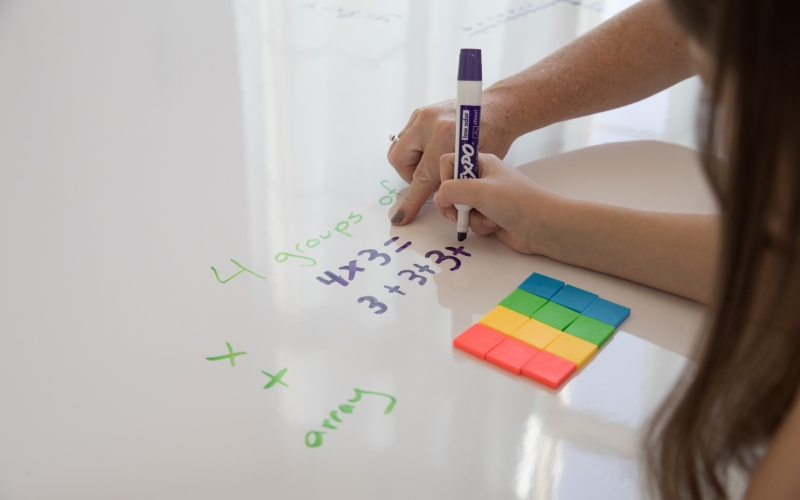Singapore Math Blog# How is Singapore math different?

Apr 26, 2021

Countries have radically different approaches to math education. But one stands out from the pack: Singapore.

This city-state has developed an approach to mathematics education that allows students to develop a real understanding of math concepts, accelerating learning, steering them away from rote learning or formula memorization.

The Singapore Ministry for Education introduced Singapore Math to the curriculum in 1982. From 1998, US practitioners began introducing it to American students in the hope that it would improve learning outcomes.

The program uses the CPA (concrete-pictorial-abstract) approach to mathematics education. It begins with physical objects and then moves towards abstract concepts gradually, building deep intuition along the way.

## The Key Differences

In the traditional US Common Core math curriculum, students learn by observing teacher examples and then repeating the method to solve further problems. Unfortunately, this approach can lead to sub-standard learning. Students don't gain a genuine understanding of the processes behind what they are doing, preventing them from applying their new knowledge in diverse contexts.

Singapore Math attempts to correct this by building knowledge conceptually. It avoids learning by analogy, replacing it with a deep understanding of mathematical principles, built from the ground up.

Students begin with real, tangible objects, such as blocks, beads, or pieces of fruit - the concrete phase of learning. They then move onto pictorial representations, such as pictures of bananas, and then use these to solve problems instead. For more advanced concepts, such as ratios and percentages, Singapore Math uses bar charts and columns, pictorially demonstrating what happens when students perform a calculation.

The final stage is the abstract one, where students learn how to manipulate mathematical notation without any references to the external world. It is only at this stage that they become familiar with operators and symbols, whereas, on the standard Common Core curriculum, they must use them from the start.

Singapore Math Dimensions is an age-appropriate syllabus following the Singapore approach. The goal of the program is to empower students to think mathematically, avoid following rote rules, and apply their knowledge inside and outside of the classroom. Dimensions Math contains elements that better serve US teachers with helpful guided instruction. These help them create an Americanized version of the original program.

## The Components Of Singapore Math

The basic components of the Singapore Math approach look similar to the regular Common Core curriculum. However, there are important differences.

Singapore Math textbooks, for instance, explicitly build on prior learning. They tend to contain fewer topics and cover them more deeply.

Singapore workbooks follow the textbooks. Like Common Core problem books, they pose problems related to the topics covered in lessons. However, they cleverly progress the difficulty of the problems, allowing the student to build math skills without requiring any excessive jumps in understanding. Most children on the course should be able to go from one problem set to another fairly rapidly.

## Wrapping Up

The basic difference between the Singapore Math approach and the regular US Common Core comes down to the focus on conceptual understanding. Singapore Math is not about memorizing formulas, but learning concepts from the ground up and then applying them to novel problems.